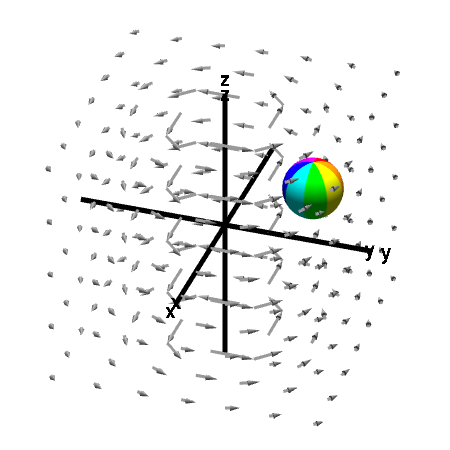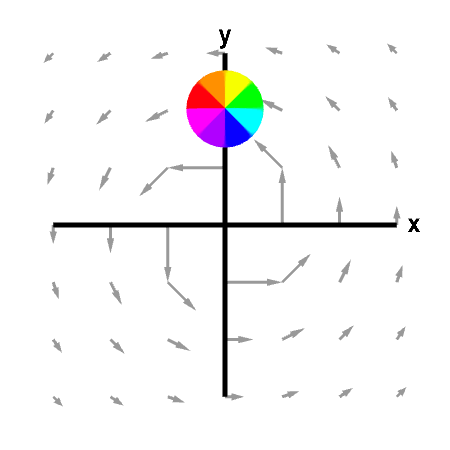# Math Insight

### Applet: A nonrotating sphere indicating absence of curlThe sphere does not rotate when its centered is fixed, indicating that, away from the $z$-axis, the vector field has no microscopic circulation, i.e., its curl is zero. On the other hand, the macroscopic circulation of the vector field around the $z$-axis is evident from the graph. This example illustrates that one cannot infer curl from the macroscopic circulation of the vector field. Macroscopic and microscopic circulation can be very different. First panel shows the full vector field; second panel shows its projection in the $xy$-plane.

This vector field is $$\dlvf(x,y,z) = \frac{(-y,x,0)}{x^2+y^2}$$ for $(x,y) \ne (0,0)$. Since for $(x,y) \ne (0,0)$, $$\pdiff{\dlvfc_2}{x} =\pdiff{\dlvfc_1}{y}= \frac{y^2-x^2}{(x^2+y^2)^2}$$ one can compute that away from the $z$-axis, $\curl \dlvf(x,y,z) = (0,0,0)$.

You can move the spheres with the mouse, though they are constrained away from the $z$-axis, where the vector field is not defined.